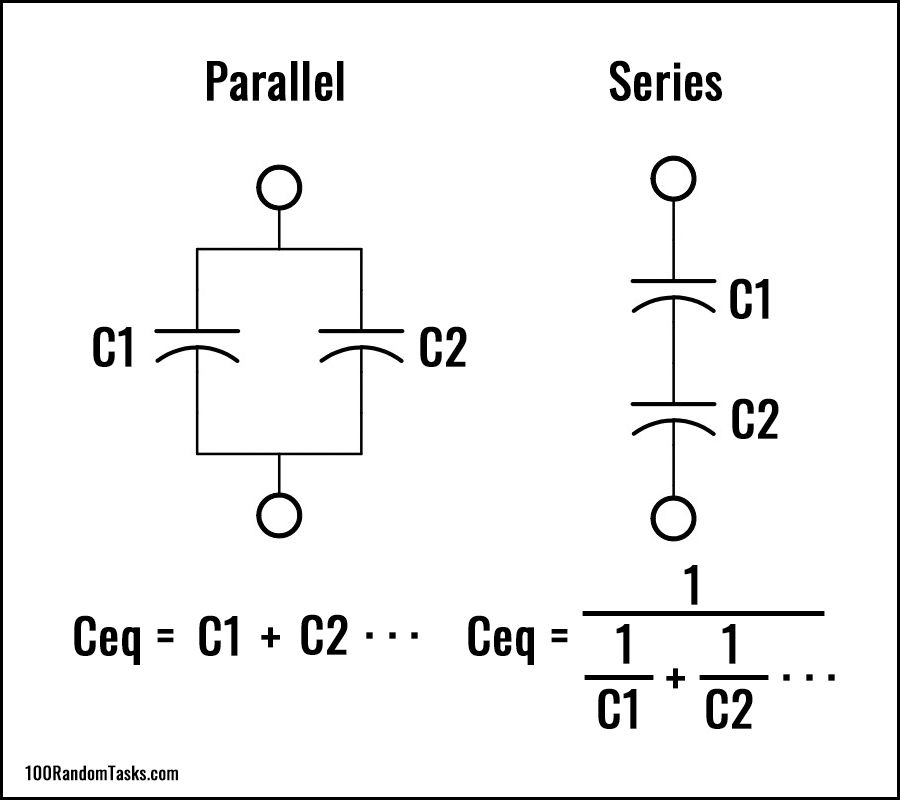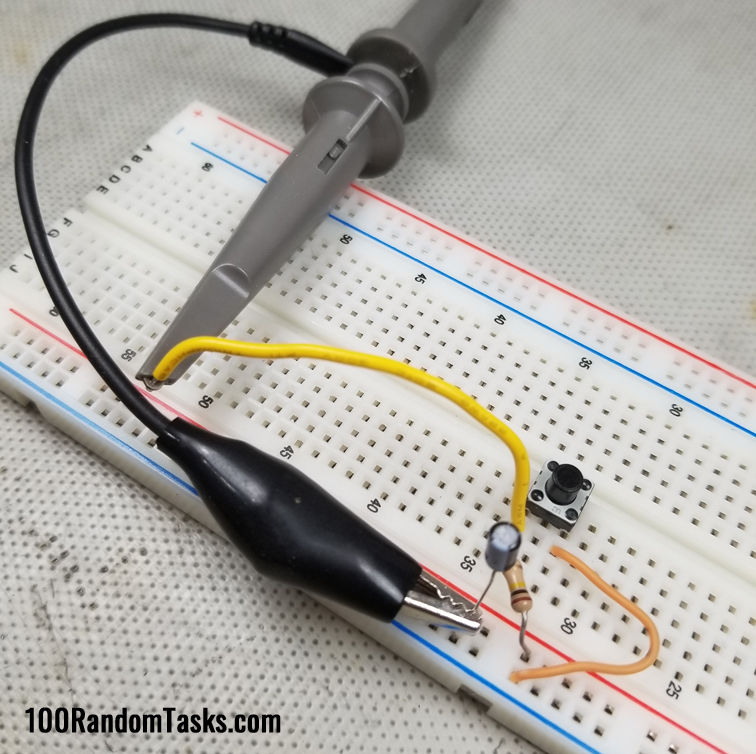top of page
Search

# HOW DO CAPACITORS WORK IN DC CIRCUITS - COFFEE BREAK

This is a first in a series of short articles describing some aspect of electronics. The first being how capacitors act in a DC environment.

So let's begin with what happens when you put capacitors in series and parallel.

## SERIES AND PARALLEL EQUIVALANTHere we have the 2 scenarios and their formulas for equivalent capacitance.

In parallel it is a straight addition and in series it is 1 over the sum of 1 over the capacitances.

This is in contrast to resistors which are the exact opposite.

Below you can see a couple of examples of actual measurements using 2 - 100uF electrolytic capacitors. From this you can see that the parallel are added, and the series are halved.## CHARGE/DISCHARGE PROFILE

All capacitors follow a defined charge and discharge profile. A graph of this profile is shown below.This profile demonstrates the amount of charge on a capacitor as a percent of maximum voltage versus time in time constants.

The time constant is simply the capacitance x the resistance the capacitor sees with the result in seconds (R x C) which will be shown in more detail below.

Things to note from this graph is that a capacitor will never reach full charge or discharge to 0. However after 5 time constants it can be assumed to be fully charged as it reaches 99.3% charge or discharged to 0.7%.

Another notable number is that capacitors will charge or discharge 63% after 1 time constant. This is useful because up to the first time constant the graph is fairly linear and can be used in ramp generators.

## CALCULATE INSTANTANEOUS TIME/VOLTAGE

There is a handy formula for calculating the instantaneous voltage across a capacitor and with a little algebra, can be rearranged to find the time it takes to get to that voltage. The added bonus to this particular formula is that the capacitor voltage can be at any initial voltage. Calculations for both voltage and time are below:v is the instantaneous voltage across the capacitor

Vf is the final voltage if the capacitor was left to charge/discharge completely

vo is the initial voltage on the capacitor.

t is time in seconds.

Tau is the time constant (R x C) in seconds.

Here you can experiment with the formulas above. The Start voltage is the initial voltage across the cap.

R and C are the values for the time constant.

The final voltage is the voltage the cap can charge/discharge to if left to fully charge/discharge.

The Calculate Voltage box will determine the voltage across the cap after Time seconds.

The Calculate Time box will determine the time required to get to End volts. Remember End volts cannot be greater than Final volts and needs to be just less than Final volts.

## VOLTAGE/CURRENT RELATIONSHIP

Finally if you haven't realized, when the capacitor is discharged and a voltage applied, the capacitor initially looks like a dead short and current is the highest and is limited by the resistor but as the capacitor charges, the voltage across it increases thereby reducing the voltage across the resistor in turn reducing the current flowing through it. From ohm's law:

I=V/R

so as V decreases, I must also decrease. Once the capacitor is fully charged, then the full voltage is across it leaving the resistor with 0 volts. With 0 across the resistor, that then makes the current 0.

With the current at 0, that makes the capacitor look to be an open. The take home message is when a capacitor begins to charge it looks like a short circuit, and when fully charged it looks like an open circuit. This can also be seen in the charge graph above. If the capacitor is charging or discharging, then the current is the same as the discharge graph.

## PRACTICAL EXAMPLE

To demonstrate this, I have created a simple circuit consisting of a 100k resistor and 1uF capacitor in series with a momentary button across the capacitor to discharge it. I have also connected an oscilloscope across the capacitor to monitor its voltage over time.The time constant for this circuit is

100k x 1uF = 0.1 Seconds or 100mS.

The current flows through the resistor to charge the capacitor.

When the button is pushed, the capacitor will discharge through the switch. Since this circuit is only using 5 volts I don't have a discharge resistor. So it will discharge almost instantly since the time constant approaches 0 because the resistance approaches 0 ohms. If the voltage was higher it could burn out the switch since current is highest at initial contact. When using higher voltages, it is important to include a discharge resistor to prevent damage. And the discharge time constant will be the capacitance x the discharge resistance.The demonstration circuit from above is shown on a breadboard with the scope probe connected.

I have my oscilloscope set up for single shot and I'll hold the capacitor at 0 volts by pressing and holding the switch while I arm the scope.

Then I'll release the switch and the scope will capture the resulting rise in voltage across the capacitor.You can see the capture here and the curve matches the charge curve from above albeit shortened in the X axis . The applied voltage in this case about 4.64V.

With a time constant of 100mS, we can see that the capacitor has charged to about 3V after the first time constant. The theoretical value should be 63% of Vcc, which is:

0.63 * 4.64V = 2.92V

Almost exactly as predicted. The other thing of note is that after 5 time constants (500mS) the voltage has climbed 4.64V also as predicted since it will reach 99.3% of max value in that time.

## CONCLUSION

I hope that this article has shed some light on how a capacitor acts in a dc circuit. With this knowledge the idea is that you will have a better understanding of the time and voltage characteristics and how they can affect each other.

Don't forget to signup for notifications of future posts and check out my YouTube channel and subscribe there for future video notifications.